Author: Oscar Cronquist Article last updated on February 10, 2023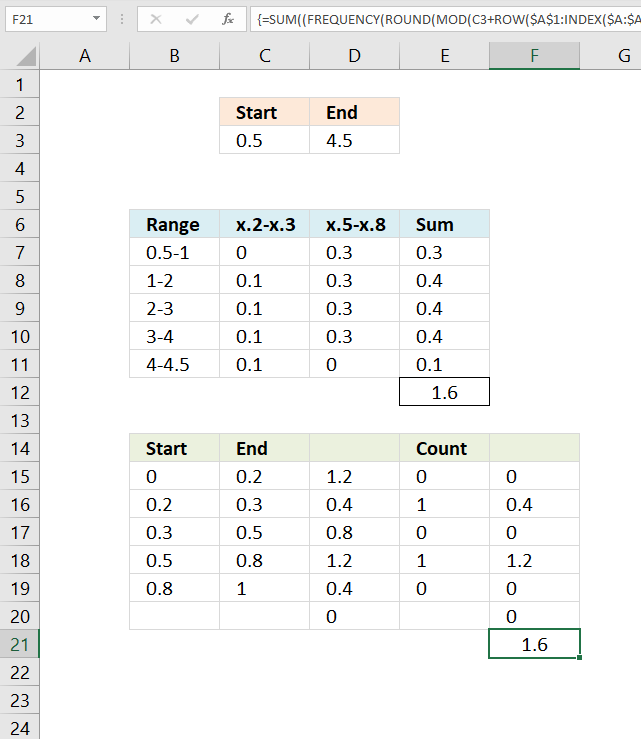This article explains how to build an array formula that sums numerical ranges. Example, I want to know how to calculate the total sum between x.2 -x.3 and x.5-x.8, and the range is 0.5 and 4.5.

Why is this useful? Excel date and time values are numerical values formatted as date/time. The last section demonstrates how to calculate a total based on time ranges and a start/end date/time.

## 1. How to manually sum ranges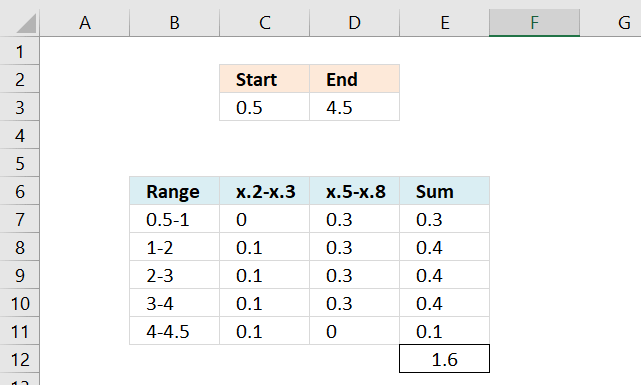This picture shows you how to manually sum the ranges x.2-x.3 and x.5-x.8 between 0.5 and 4.5.

The range starts at 0.5 so 0.2-0.3 is 0. 0.5-0.8 is  0.3. Total sum between 0.5-1 is 0.3.
1.2-1.3 is 0.1 and 1.5-1.8 is 0.3. The total between 1-2 is 0.4.
2.2-2.3 is 0.1 and 2.5-2.8 is 0.3. The total between 2-3 is 0.4.
3.2-3.3 is 0.1 and 3.5-3.8 is 0.3. The total between 3-4 is 0.4.
4.2-4.3 is 0.1 and 4.5-4.8 is 0 because the range ends at 4.5. The total between 4-4.5 is 0.1.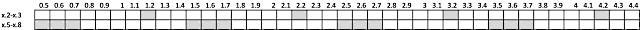The grand total is 1.6 (0.3+0.4+0.4+0.4+0.4+0.1 = 1.6).

## 2. How to sum ranges in ExcelArray formula in cell F21:

=SUM((FREQUENCY(ROUND(MOD(C3+ROW(\$A\$1:INDEX(\$A:\$A, (D3-C3)*10))/10, 1), 1), \$C\$15:\$C\$19)/10)*E15:E20)

#### Step 1 - Create cell reference

The INDEX function is able to create a cell reference that can be used to create an array. This technique is better than using the INDIRECT function which is volatile.

INDEX(\$A:\$A, (D3-C3)*10)

becomes

INDEX(\$A:\$A, (4.5-0.5)*10)

becomes

INDEX(\$A:\$A, 4*10)

becomes

INDEX(\$A:\$A, 40)

and returns A40

#### Step 2 - Create a cell reference to a cell range

You can concatenate the output from the INDEX function with another cell reference so that it points to a cell range. Weirdly, you don't need to use the ampersand character or double quotations to do so. This is the only case that I know of when this is possible.

\$A\$1:INDEX(\$A:\$A, (D3-C3)*10)

becomes

\$A\$1:A40

#### Step 3 - Create an array based on a cell reference

The ROW function returns a number representing the row number from a cell reference. The ROW function returns an array of row numbers ff the cell reference points to a cell range.

ROW(\$A\$1:INDEX(\$A:\$A, (D3-C3)*10))

becomes

ROW(\$A\$1:A40)

and returns {1; 2; 3; 4; 5; 6; 7; 8; 9; 10; 11; 12; 13; 14; 15; 16; 17; 18; 19; 20; 21; 22; 23; 24; 25; 26; 27; 28; 29; 30; 31; 32; 33; 34; 35; 36; 37; 38; 39; 40}

#### Step 4 - Divide by 10

ROW(\$A\$1:INDEX(\$A:\$A, (D3-C3)*10))/10

becomes

{1; 2; 3; 4; 5; 6; 7; 8; 9; 10; 11; 12; 13; 14; 15; 16; 17; 18; 19; 20; 21; 22; 23; 24; 25; 26; 27; 28; 29; 30; 31; 32; 33; 34; 35; 36; 37; 38; 39; 40}/10

and returns

{0.1; 0.2; 0.3; 0.4; 0.5; 0.6; 0.7; 0.8; 0.9; 1; 1.1; 1.2; 1.3; 1.4; 1.5; 1.6; 1.7; 1.8; 1.9; 2; 2.1; 2.2; 2.3; 2.4; 2.5; 2.6; 2.7; 2.8; 2.9; 3; 3.1; 3.2; 3.3; 3.4; 3.5; 3.6; 3.7; 3.8; 3.9; 4}

#### Step 5 - Create a sequence

C3+ROW(\$A\$1:INDEX(\$A:\$A, (D3-C3)*10))/10

becomes

C3+{0.1; 0.2; 0.3; 0.4; 0.5; 0.6; 0.7; 0.8; 0.9; 1; 1.1; 1.2; 1.3; 1.4; 1.5; 1.6; 1.7; 1.8; 1.9; 2; 2.1; 2.2; 2.3; 2.4; 2.5; 2.6; 2.7; 2.8; 2.9; 3; 3.1; 3.2; 3.3; 3.4; 3.5; 3.6; 3.7; 3.8; 3.9; 4}

becomes

0.5+{0.1; 0.2; 0.3; 0.4; 0.5; 0.6; 0.7; 0.8; 0.9; 1; 1.1; 1.2; 1.3; 1.4; 1.5; 1.6; 1.7; 1.8; 1.9; 2; 2.1; 2.2; 2.3; 2.4; 2.5; 2.6; 2.7; 2.8; 2.9; 3; 3.1; 3.2; 3.3; 3.4; 3.5; 3.6; 3.7; 3.8; 3.9; 4}

and returns

{0.6; 0.7; 0.8; 0.9; 1; 1.1; 1.2; 1.3; 1.4; 1.5; 1.6; 1.7; 1.8; 1.9; 2; 2.1; 2.2; 2.3; 2.4; 2.5; 2.6; 2.7; 2.8; 2.9; 3; 3.1; 3.2; 3.3; 3.4; 3.5; 3.6; 3.7; 3.8; 3.9; 4; 4.1; 4.2; 4.3; 4.4; 4.5}

#### Step 6 - Filter decimals from number

We only need the decimal part of the values in this array.

MOD(C3+ROW(\$A\$1:INDEX(\$A:\$A, (D3-C3)*10))/10, 1)

becomes

MOD({0.6; 0.7; 0.8; ... ; 4.3; 4.4; 4.5}, 1)

and returns

{0.6;0.7;0.8; ... ;0.2;0.3;0.4;0.5}

#### Step 7 - Workaround for floating point error

ROUND(MOD(C3+ROW(\$A\$1:INDEX(\$A:\$A, (D3-C3)*10))/10, 1), 1)

ROUND({0.6;0.7;0.8; ... ;0.2;0.3;0.4;0.5}, 1)

and returns  {0.6; 0.7; ... 0.3; 0.4; 0.5}.

The MOD function returns the decimal part of a number, unfortunately, it returns a floating point error. The ROUND function takes care of that.

#### Step 8 - Evaluate FREQUENCY function

The second task is to build the ranges. We are going to use the FREQUENCY function and therefore we need to be more specific regarding the ranges.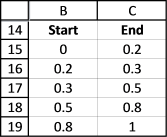The picture shows not only 0.2-0.3 and 0.5-0.8 but also other ranges before, between, and after. We are not interested in those ranges but they are required in order to get the FREQUENCY function to work as we want.

It is now time to use the frequency function with our array and our ranges:

=FREQUENCY(ROUND(MOD(C3+ROW(\$A\$1:INDEX(\$A:\$A, (D3-C3)*10))/10, 1), 1), \$C\$15:\$C\$19)/10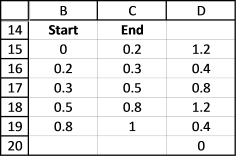The array formula returns an array shown in D15:D20. The range we are using is 0.5 to 4.5, remember? 4.5 - 0.5 is 4. 4 is supposed to be equal to the sum of the frequency values in D15:D20. Lets verify that, 1.2+0.4+0.8+1.2+0.4 = 4. Correct.

#### Step 9 - Multiply arrays

The third task is to sum the ranges we need. In order to do that I have built a new column "Count", 1 for a range I want in the sum and 0 for a range I don´t want.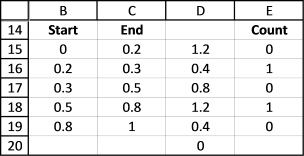Lets multiply the array formula with the "Count" column (E15:E19):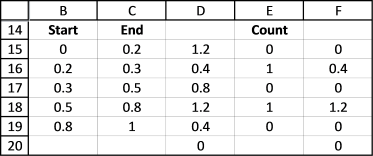=(FREQUENCY(ROUND(MOD(C3+ROW(\$A\$1:INDEX(\$A:\$A, (D3-C3)*10))/10,1),1), \$C\$15:\$C\$19)/10)*E15:E20

This formula returns {0; 0.4; 0; 1.2; 0; 0}.

Then use the SUM function to sum the values in the array:

=SUM((FREQUENCY(ROUND(MOD(C3+ROW(\$A\$1:INDEX(\$A:\$A, (D3-C3)*10))/10,1),1), \$C\$15:\$C\$19)/10)*E15:E20)

returns 1.6. 0.4 + 1.2 is 1.6, it matches the manual calculation we did in the beginning of this blog post.

Why would you want to sum numerical ranges? Consider that date and time values are actually numbers in excel, get it? Read the next section below.

## 3. How to sum time based on time periods across days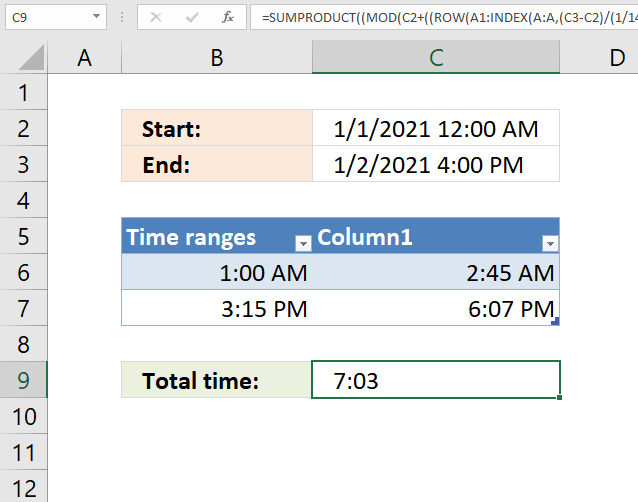The array formula in cell C9 is adapted to counting minutes, it compares the time range based on the values in cell C2 and C3 (start and end date/time) to the time ranges in the Excel Table (cell range B6:C7) and adds the minutes that fall between the time ranges.

You can easily add more time ranges to the Excel Table without adjusting the formula. There are two structured references that point to the Excel Table, Table1[Column1] and Table1[Time ranges].

The array formula in cell C9 recalculates if you change the start/end value in C2,C3 or add/delete/edit the time ranges in B6:C7. Note that there is a limit to the size of the start and end date, the formula won't work if the range is larger than 728,18 days. This is because the array size in Excel formulas is limited to 1048576 values.

Array formula in cell C9:

=SUMPRODUCT((MOD(C2+((ROW(A1:INDEX(A:A, (C3-C2)/(1/1440)))-1)/1440), 1)<TRANSPOSE(Table1[Column1]))*(MOD(C2+((ROW(A1:INDEX(A:A, (C3-C2)/(1/1440)))-1)/1440), 1)>=TRANSPOSE(Table1[Time ranges])))/(24*60)

Excel 365 formula in cell C9

=LET(y, 1440,x,MOD(C2+(SEQUENCE(, (C3-C2)/(1/y))-1)/y, 1), SUMPRODUCT((x<Table1[Column1])*(x>=Table1[Time ranges]))/y)

### Get the Excel fileSum-numerical-rangesv3.xlsx